# 3.1 模板：重复可用的代码 _之 数字货币现货交易类库

Author: 小小梦, Created: 2016-11-12 11:25:51, Updated: 2020-03-21 18:53:14

• ### 认识 “模板类库”

先来认识模板（其实在 2.6 章节 我们已经初步接触到 模板了） 我们简称的“模板”，在 发明者量化 平台的策略类别分类里面叫做 “模板类库”。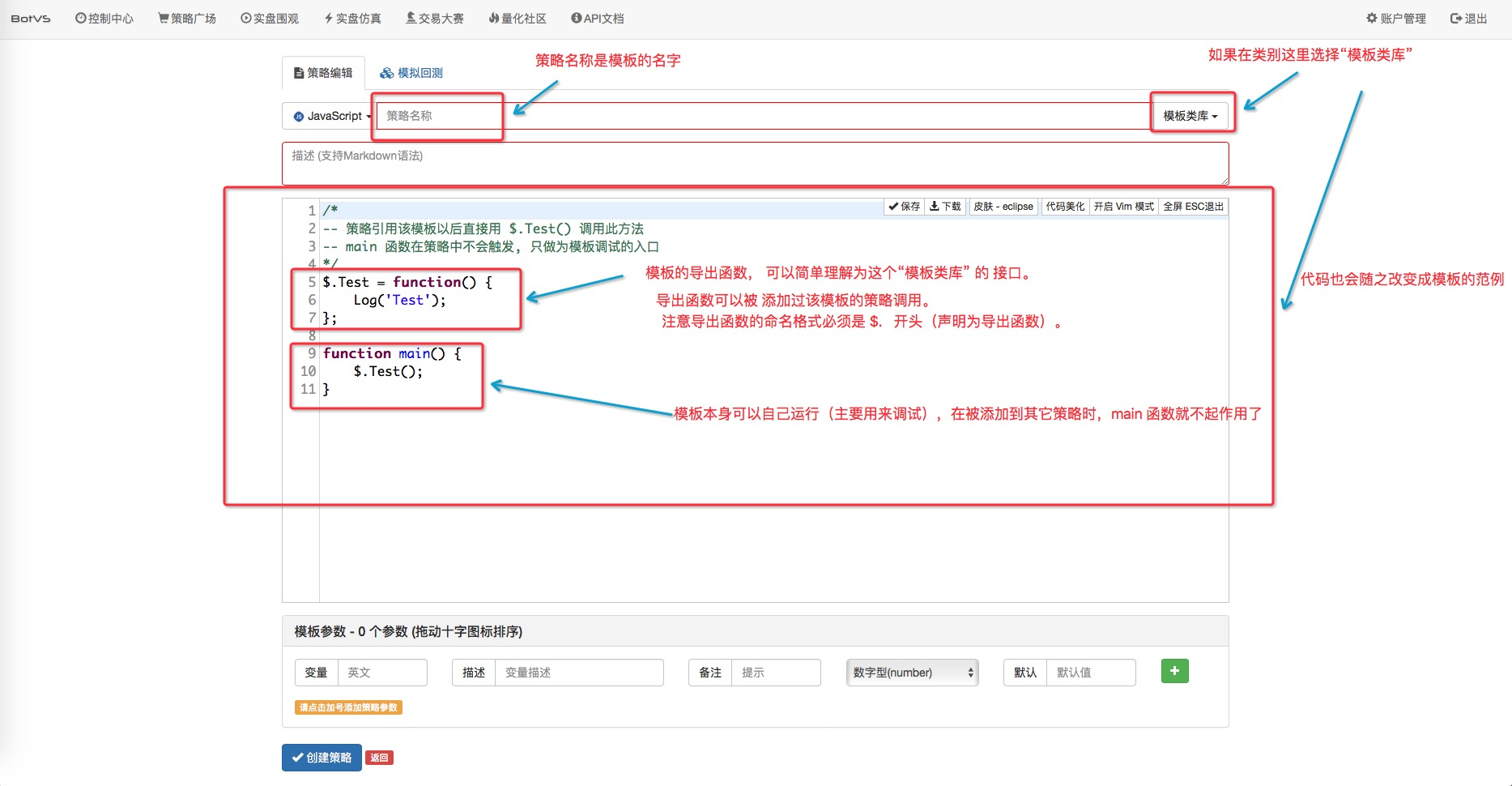导出函数的命名方式必须是 \$.functionName = function(){…}; 。 function \$.functionName(){…} 是错误的。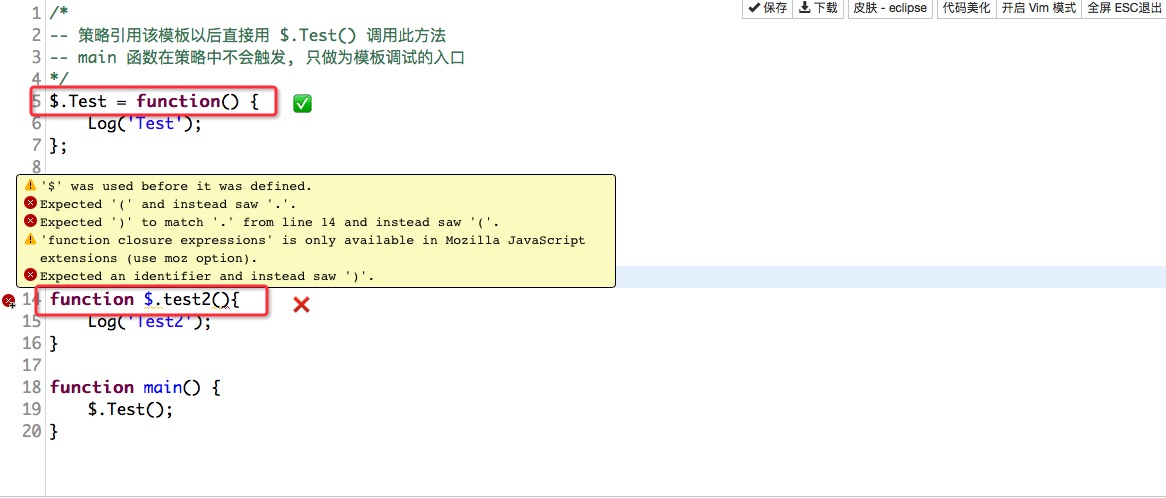我们就创建一个 名为 “测试模板” 的模板类库，如下图：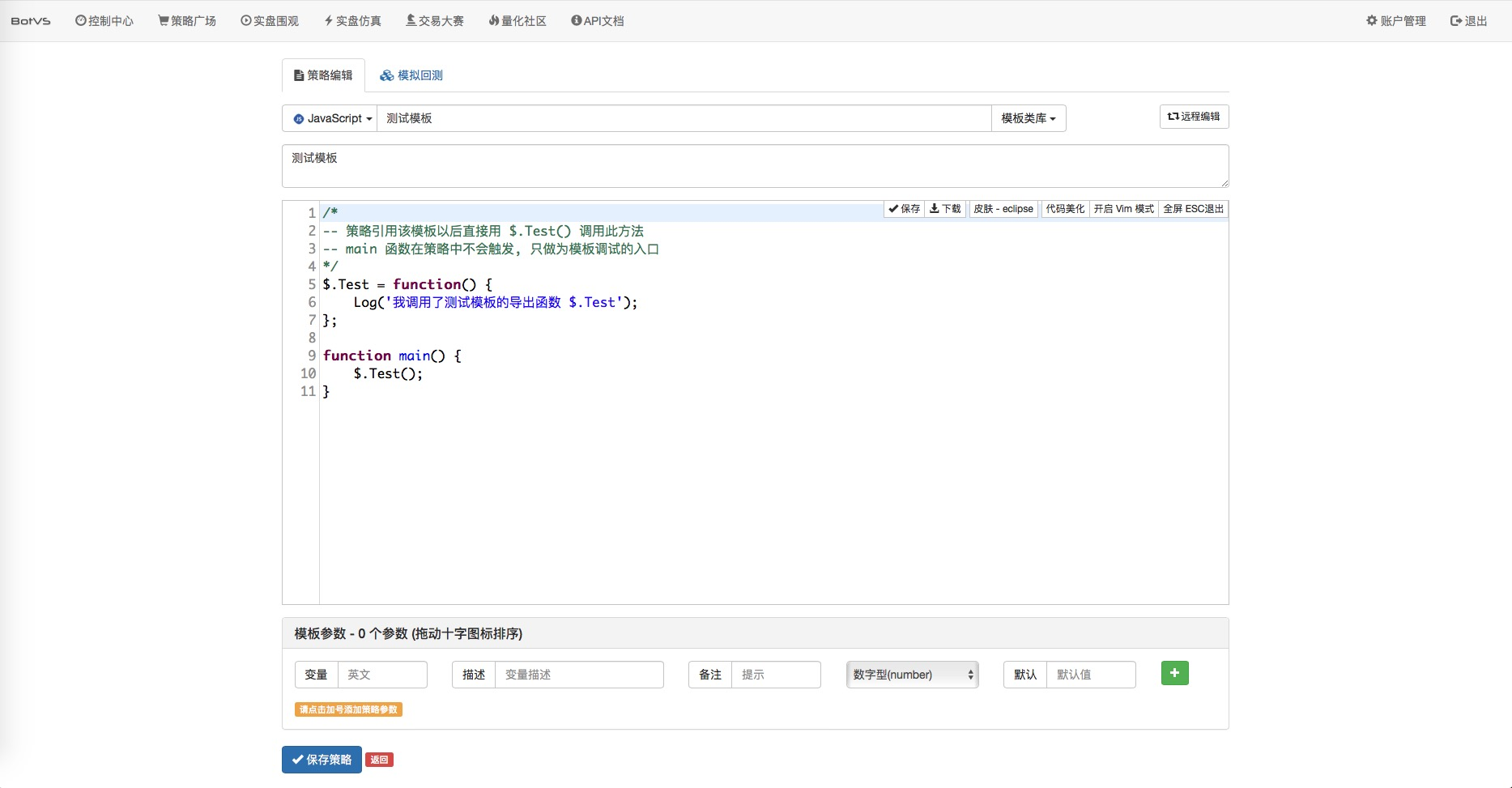进入要添加模板的策略。添加模板：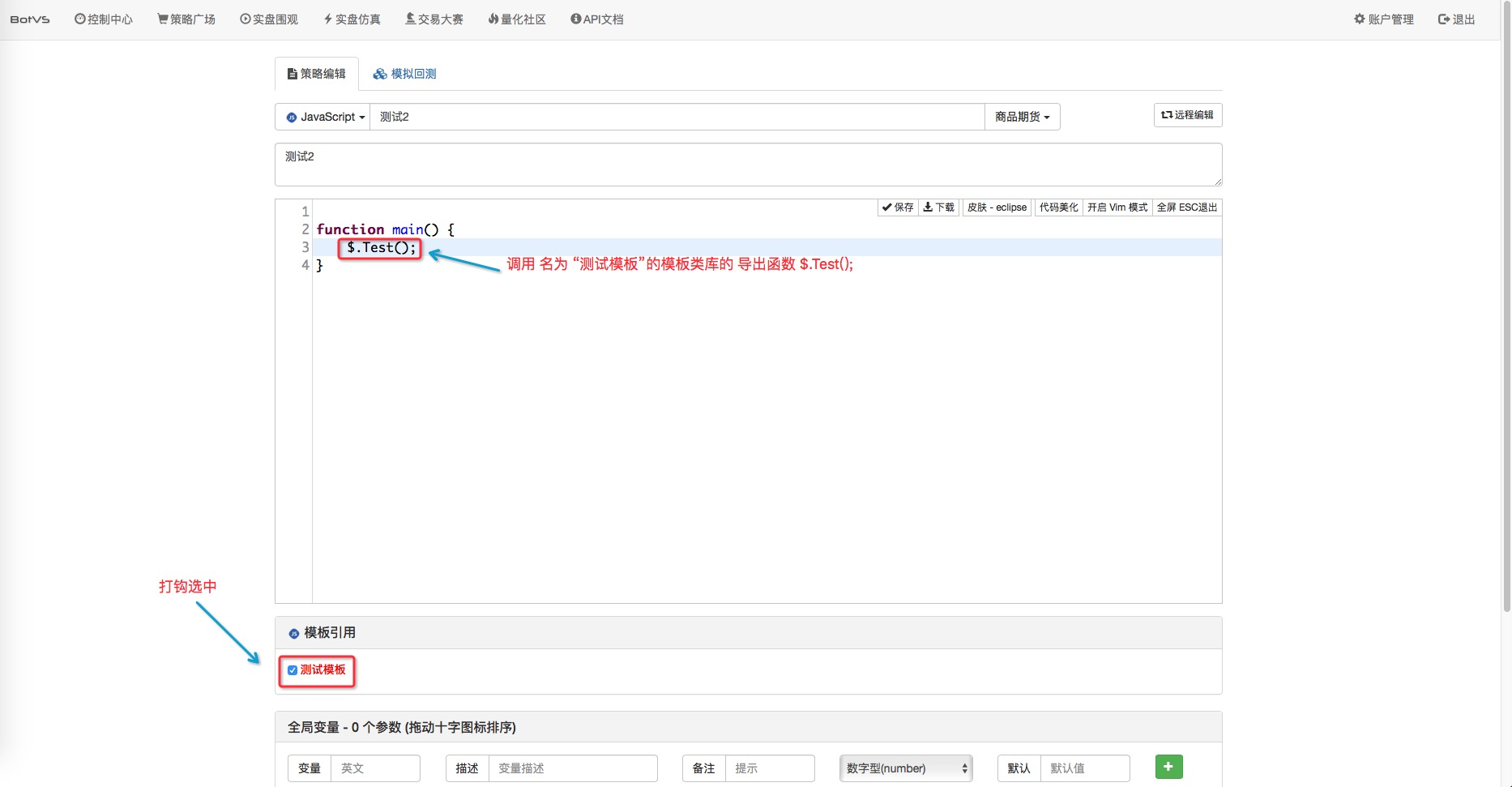一定记得保存！， 然后我们回测看看。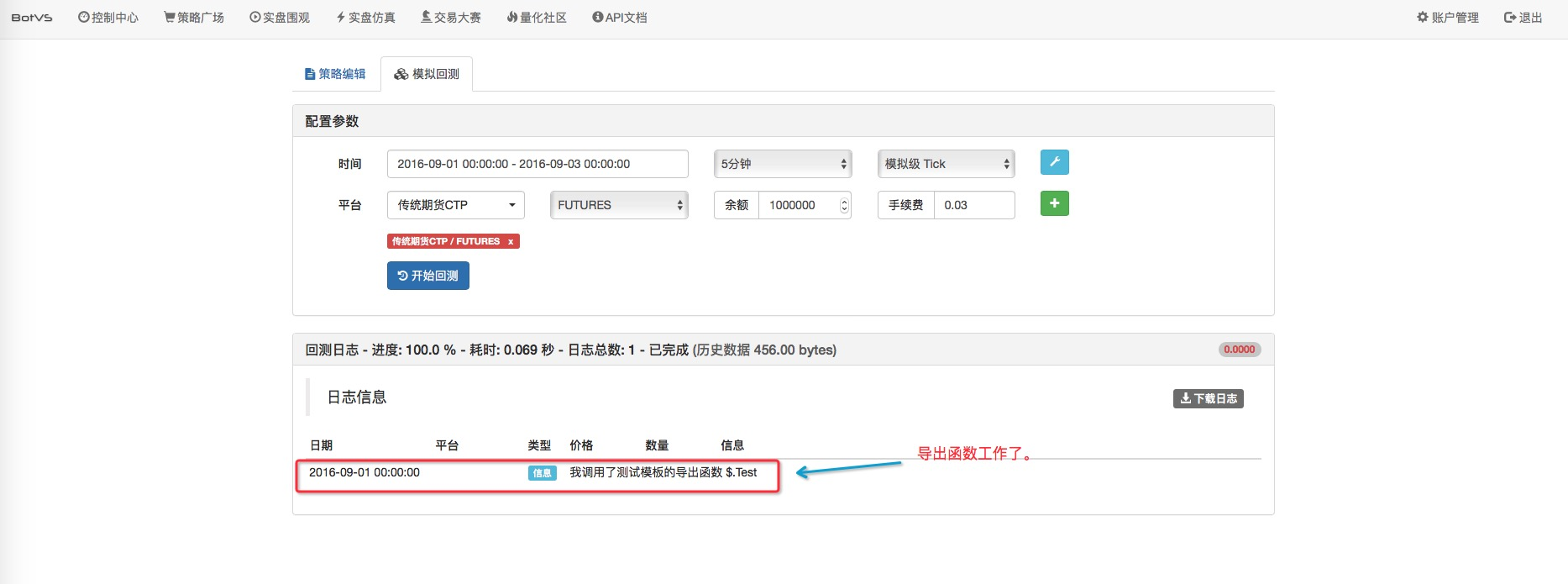• ### Python 版本的 模板

• 1、导出函数 命名

模板中 这样编写导出函数和导出函数的实现函数。

``````def test():               # 实现
Log("测试！")

ext.fun_Export = test     # 导出函数
``````

调用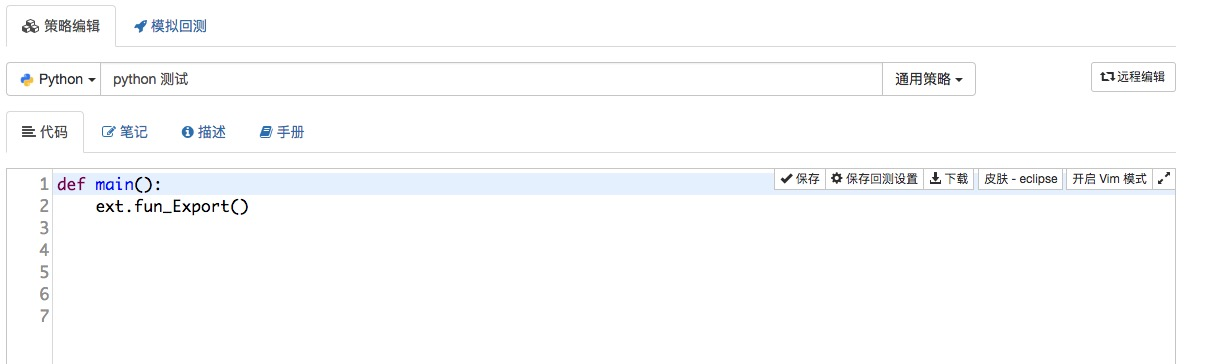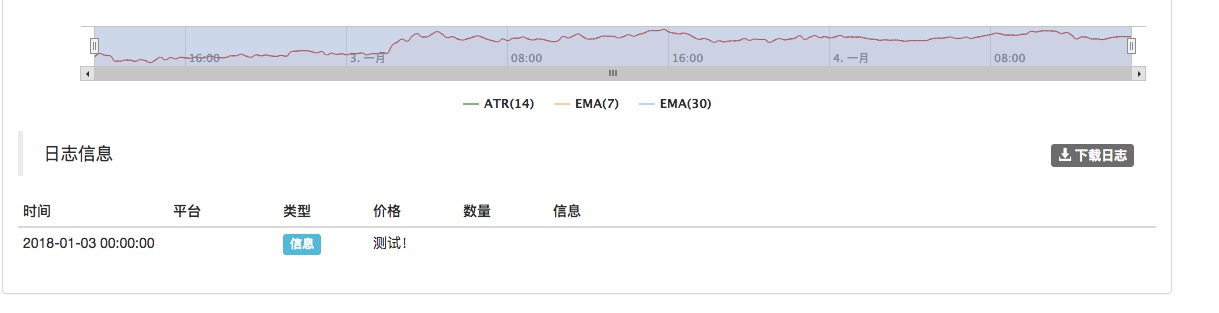• 2、添加模板

给策略引用上模板的方式 和 JS版本的模板 一样，勾选相应的模板，保存。

• ### 模板类库 之 《数字货币现货交易类库》

如果用户编程技术这方面正在学习，但是苦于对交易细节方面的把握，而又想写一些策略。这个时候可以用到 数字货币现货交易类库。该模板类库已经封装好了交易逻辑，方便使用。 推荐先通读一边代码，理解代码实现，在使用的时候才能更加得心应手，先去复制模板。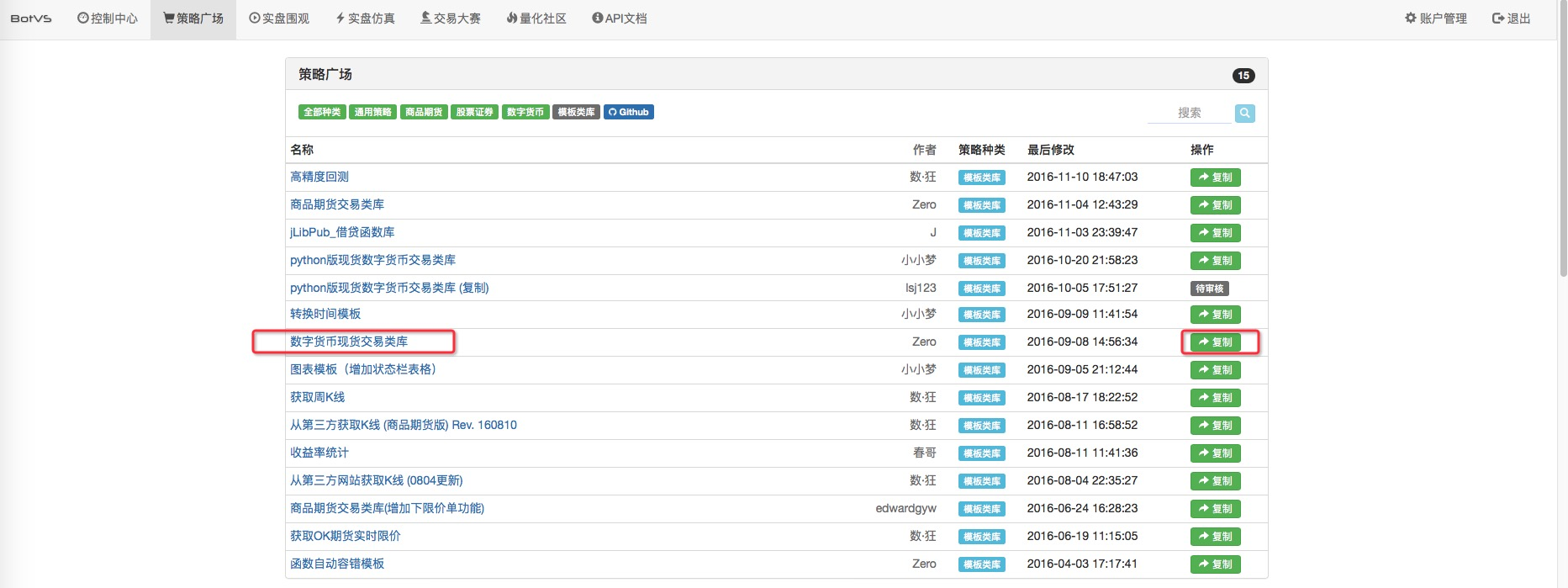添加上 数字货币现货交易类库 模板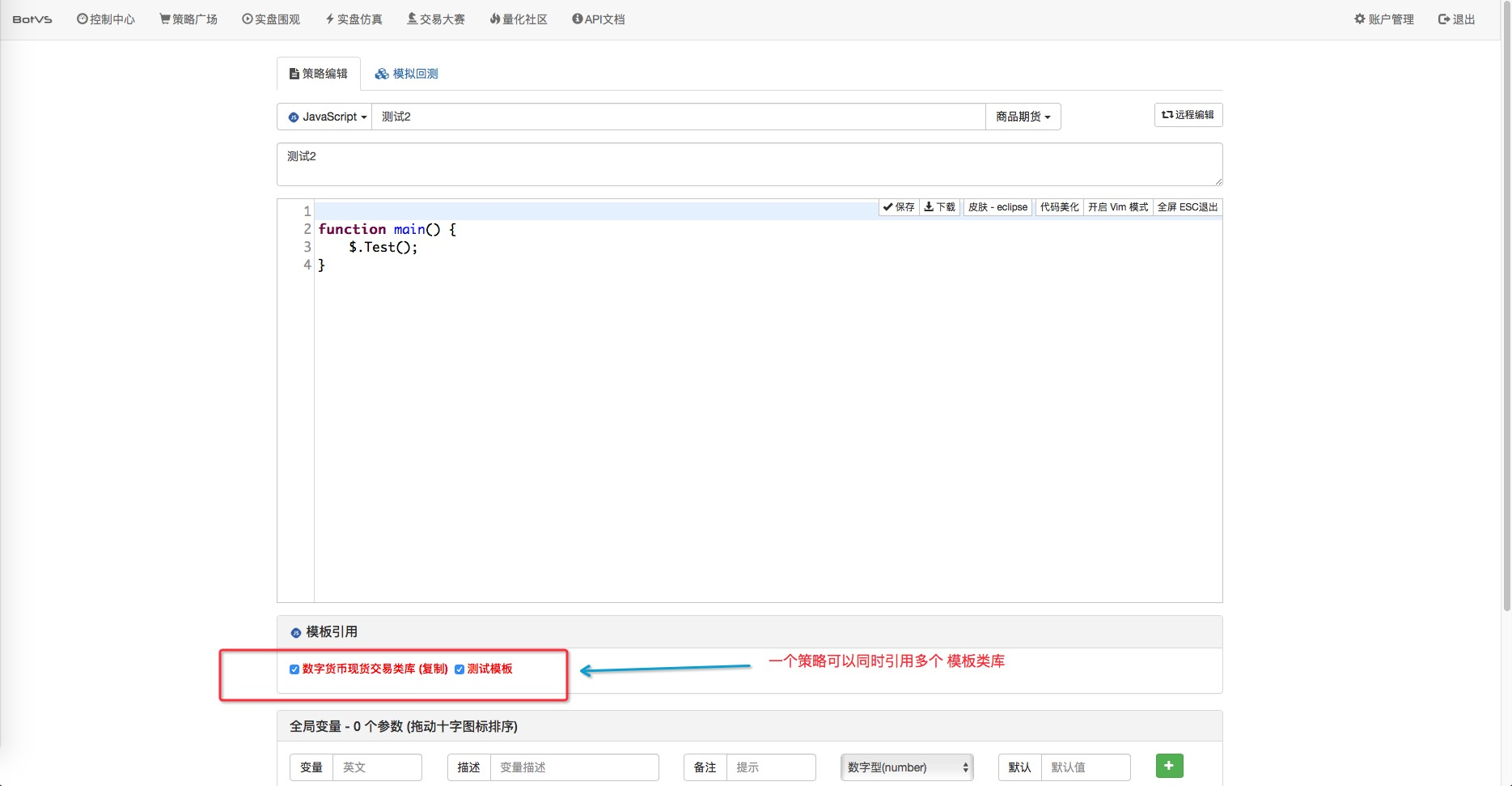接下来我们使用一下，对于该模板导出函数不清楚的同学可以自行查看该模板的源码，一会儿我们分析源码，先看使用。 还记得我们自己写的策略交互函数 get_Command() 么？ 在2.5章节 我们用这个函数只是模拟 交易（仅仅Log一下），下面我们来真实的下单测试，选择 发明者量化 模拟盘作为测试交易所。

``````// 交互函数
function get_Command() { //负责交互的函数，交互及时更新 相关数值 ，熟悉的用户可以自行扩展
var keyValue = 0; // 命令传来的参数 数值
var way = null; //路由
var cmd = GetCommand(); //获取  交互命令API
if (cmd) {
Log("按下了按钮：", cmd); //日志显示
arrStr = cmd.split(":"); // GetCommand 函数返回的 是一个字符串，这里我处理的麻烦了，因为想熟悉一下JSON
//，所以先对字符串做出处理，把函数返回的字符串以 : 号分割成2个字符串。储存在字符串数组中。

if (arrStr.length === 2) { //接受的不是 数值型的，是按钮型的。
jsonObjStr = '{' + '"' + arrStr + '"' + ':' + arrStr + '}'; // 把 字符串数组中的元素重新
//拼接 ，拼接成 JSON 字符串  用于转换为JSON 对象。
jsonObj = JSON.parse(jsonObjStr); // 转换为JSON 对象

for (var key in jsonObj) { // 遍历对象中的  成员名
keyValue = jsonObj[key]; //取出成员名对应的 值 ， 就是交互按钮的值
}

if (arrStr == "") { // 此处为 数字型  。这里处理分为  按钮  和  数字型  。 详见 策略参数 设置界面 下的 交互设置
way = 1;
}
if (arrStr == "") {
way = 2;
}
if (arrStr == "扩展2") {
way = 3;
}
if (arrStr == "扩展3") {
way = 4;
}
} else if (arrStr.length === 1) { // 此处为 按钮型
//路由
if (cmd == "buy") {   //  buy 就是 添加的控件的 名称  在下图 已经用红框 圈出来了
way = 0;
}
if (cmd == "sell") {  //  sell 就是 添加的控件的 名称  在下图 已经用红框 圈出来了
way = 5;
}
} else {
throw "error:" + cmd + "--" + arrStr;
}
switch (way) { // 分支选择 操作
break;
case 1: //处理
break;
case 2: //处理
break;
case 3: //处理
break;
case 4: //处理
break;
case 5: //处理 sell 按钮
singal = SELL;
break;
default:
break;
}
}
}
var WAITING = 0;
var SELL = 2;
var singal = WAITING;
function main() {
var initAccount = _C(exchange.GetAccount);
Log("初始账户信息：", initAccount);
var sellInfo = null;
while(true){
get_Command();            // 获取交互 命令
singal = WAITING;
}
if(singal === SELL){
sellInfo = \$.Sell(0.5);
Log("nowAccount:", _C(exchange.GetAccount), "sellInfo:", sellInfo);
singal = WAITING;
}
Sleep(1000);
}
}
``````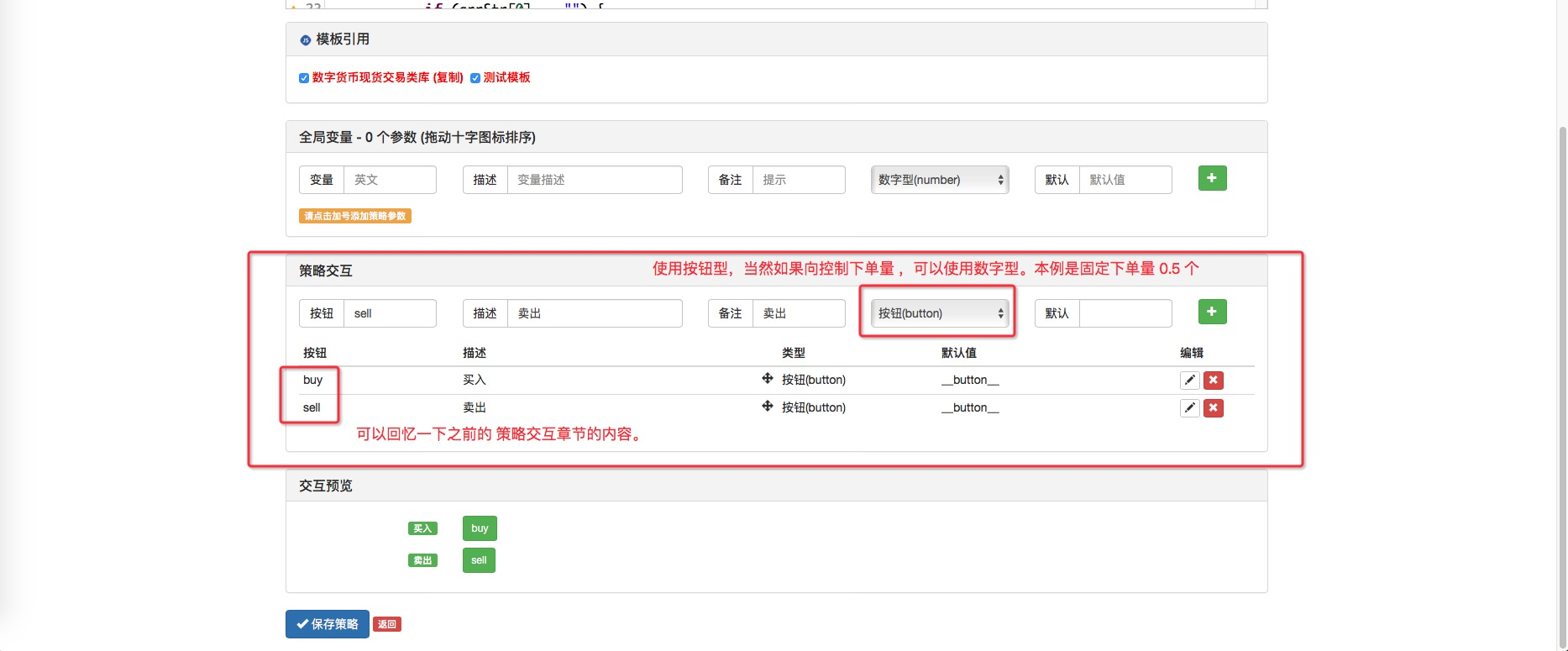OK！ 来测试一下：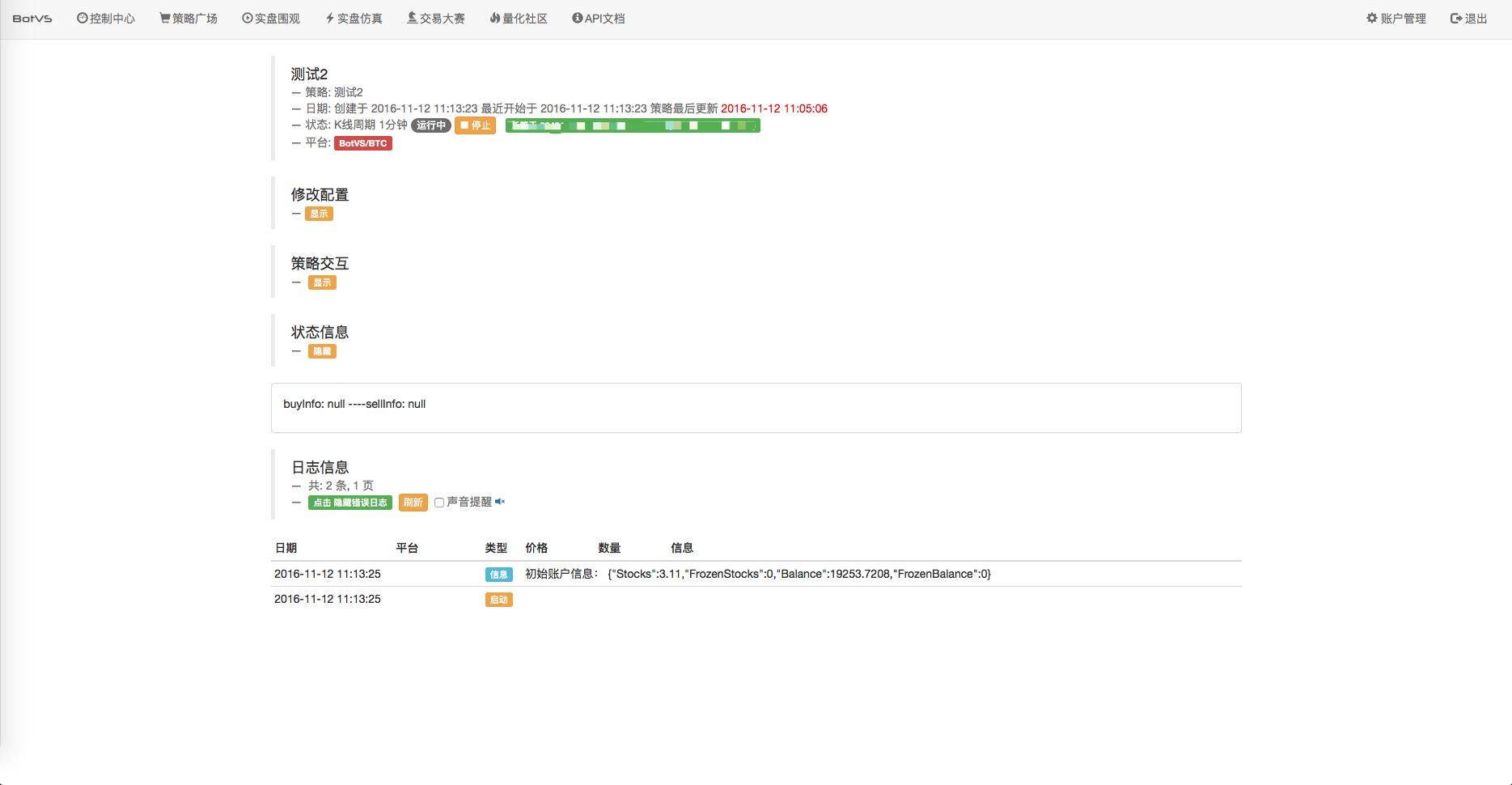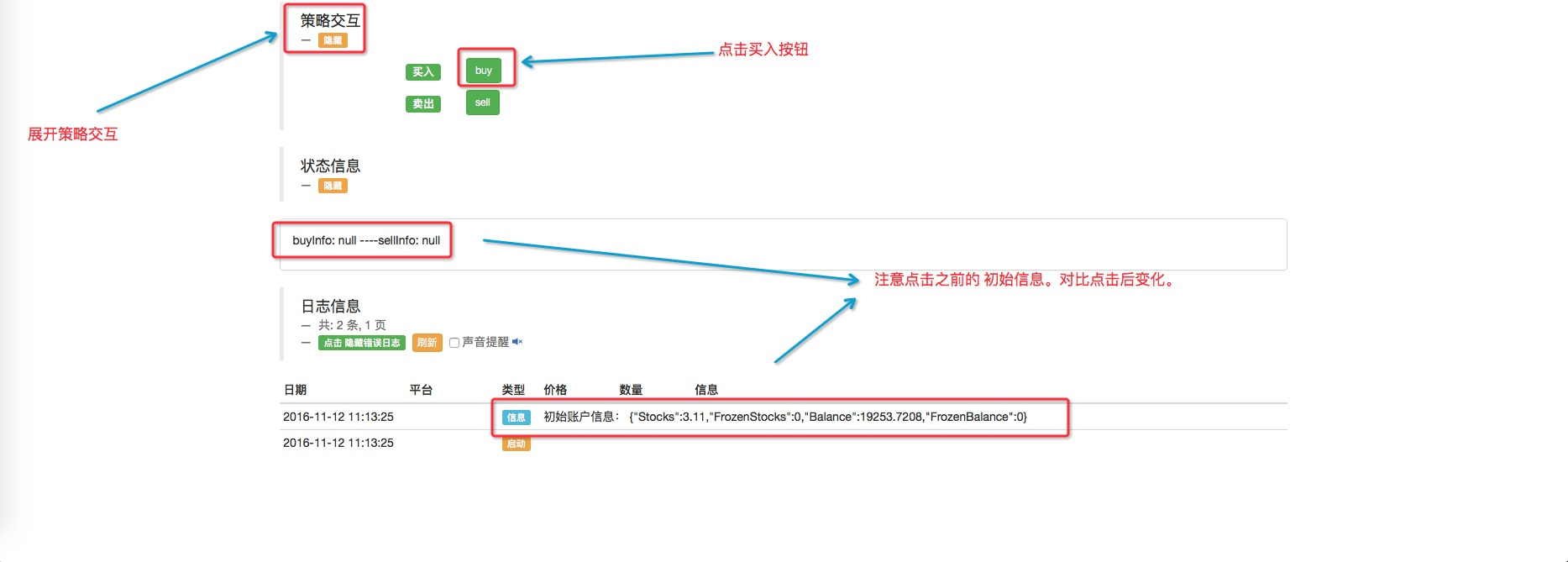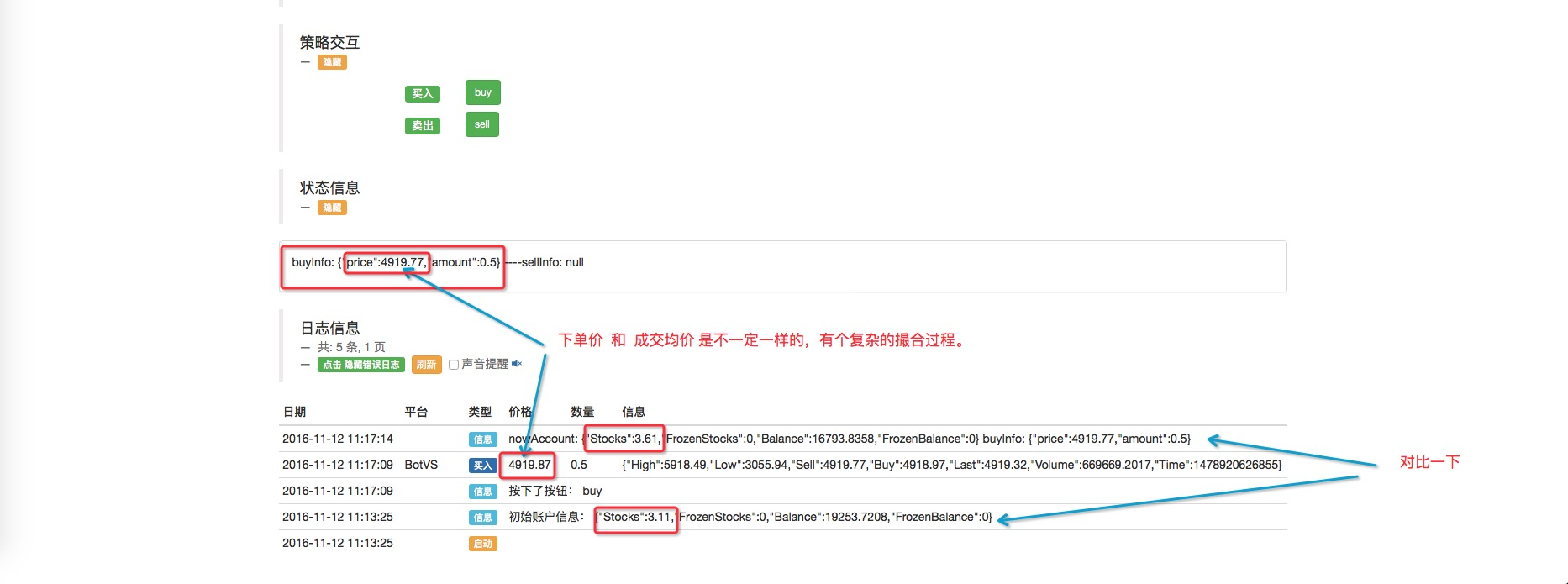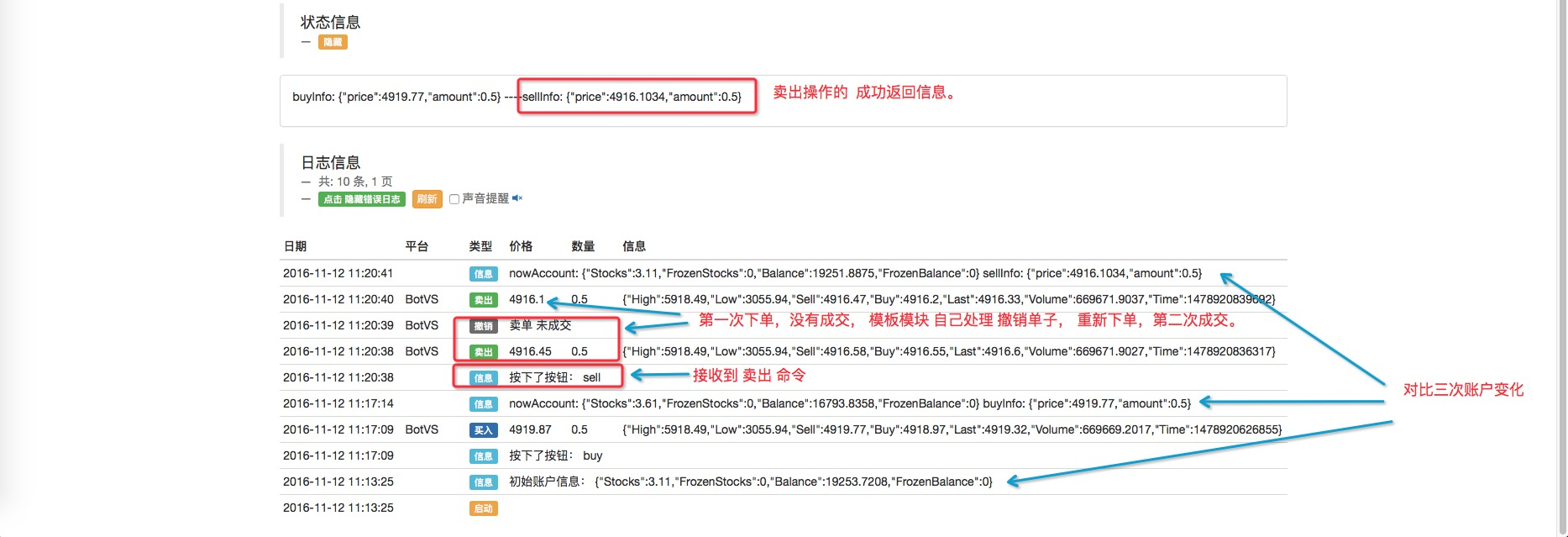• ### 剖析 数字货币现货交易类库 源码

想要用好一个工具，必须要彻底了解它。 看代码不能脱离界面参数：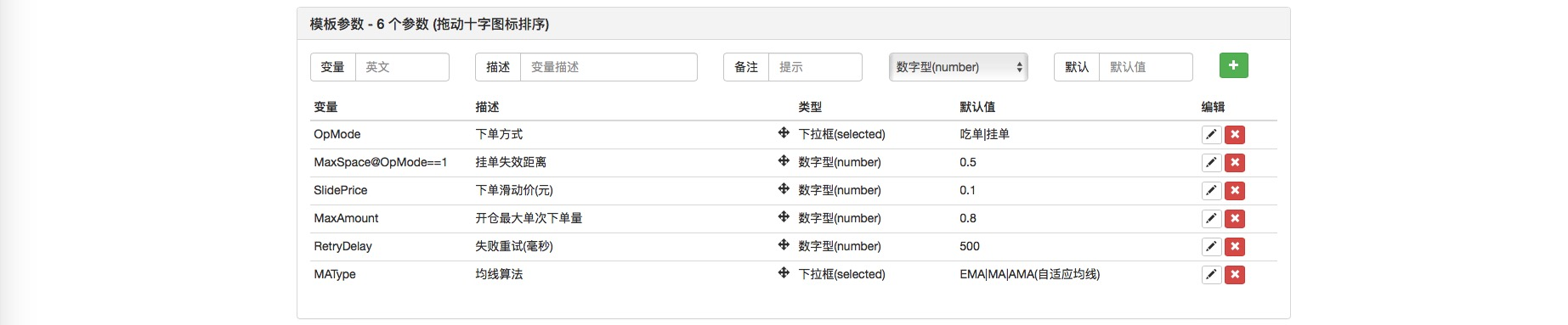源码：

``````function CancelPendingOrders(e, orderType) { // 该函数作用是 取消所有挂单
while (true) {
var orders = e.GetOrders();  //  获取 所有未成交的挂单
if (!orders) {               //  容错处理： orders 获取异常 为null 的情况。
Sleep(RetryDelay);
continue;
}
var processed = 0;           //  处理计数 ， 每次循环初始 复制为0 ， 一旦最后检测 仍然为0 即证明没有 挂单需要处理，结束while循环。
for (var j = 0; j < orders.length; j++) {  //  遍历 orders 数组，访问每一个未成交的挂单。
if (typeof(orderType) === 'number' && orders[j].Type !== orderType) {  // 如果指定了 参数orderType 只处理 orderType类型的挂单，其它跳过。
continue;
}
e.CancelOrder(orders[j].Id, orders[j]);     //  取消当前索引的挂单。
processed++;                                //  处理计数自加
if (j < (orders.length - 1)) {              //  当前索引小于 数组orders 最后一个索引时 执行Sleep
Sleep(RetryDelay);
}
}
if (processed === 0) {       //  处理计数  等于 初始值 即没有 需要处理的挂单， 跳出 while 循环。
break;
}
}
}
function GetAccount(e, waitFrozen) {  // 获取账户信息， 可以指定 是否等待冻结
if (typeof(waitFrozen) == 'undefined') {  // 如果没有传入  waitFrozen 函数， 赋值 waitFrozen 为false
waitFrozen = false;
}
var account = null;            // 声明一个变量
while (true) {
account = _C(e.GetAccount);   //  调用API 获取当前账户信息
if (!waitFrozen || (account.FrozenStocks < e.GetMinStock() && account.FrozenBalance < 0.01)) {
// 如果不等待冻结，就不判断 (account.FrozenStocks < e.GetMinStock() && account.FrozenBalance < 0.01)
// 即 符合 if 条件 执行以下 break; 语句。
break;
}
Log("发现账户有冻结的钱或币", account);   //  输出一条日志 提醒
}
Sleep(RetryDelay);
} // 注意 ： 如果有冻结的钱 或者 币  有可能一直卡在此处。
return account;  //  返回账户信息
}
function StripOrders(e, orderId) {  // 该函数在 2.4 章节 也介绍过。
var order = null;
if (typeof(orderId) == 'undefined') {
orderId = null;
}
while (true) {
var dropped = 0;
var orders = _C(e.GetOrders);
for (var i = 0; i < orders.length; i++) {
if (orders[i].Id == orderId) {
order = orders[i];
} else {
var extra = "";
if (orders[i].DealAmount > 0) {
extra = "成交: " + orders[i].DealAmount;
} else {
extra = "未成交";
}
e.CancelOrder(orders[i].Id, orders[i].Type == ORDER_TYPE_BUY ? "买单" : "卖单", extra);
dropped++;
}
}
if (dropped === 0) {
break;
}
Sleep(RetryDelay);
}
return order;
}
// 交易函数，e:交易所对象 ， tradeType:交易类型 ， tradeAmount：交易数量， mode：模式, slidePrice：滑价， maxAmount:单次最大交易量， maxSpace: 最大挂单距离， retryDelay: 重试时间。
var initAccount = GetAccount(e, true);  // 进入交易函数 初始时 获取账户信息。
var nowAccount = initAccount;           // 声明一个 用于保存当前账户信息的变量，并初始化为 initAccount
var orderId = null;                     // 声明一个用于保存 订单ID 的变量
var prePrice = 0;                       // 上一次的价格
var dealAmount = 0;                     // 已经处理过的（成交过的） 交易数量
var diffMoney = 0;                      // 账户 钱之差
var isFirst = true;                     // 是否是 第一次的循环的 标记
while (true) {  // while 循环
var ticker = _C(e.GetTicker);    // 获取当前行情数据。
// _C 不清楚的请查阅 平台论坛 相关帖子https://www.fmz.com/bbs-topic/320
var tradePrice = 0;              // 初始交易价格 0
if (isBuy) { // 如果是 买入操作
tradePrice = _N((mode === 0 ? ticker.Sell : ticker.Buy) + slidePrice, 4); // 根据挂单模式 还是吃单模式，去计算下单价格， mode 为 当前函数的参数。
// 对于 _N 不清楚的可以查询 https://www.fmz.com/bbs-topic/320 第7个问题。
} else {
tradePrice = _N((mode === 0 ? ticker.Buy : ticker.Sell) - slidePrice, 4);
}
if (!orderId) { // 判断 是否已经下单，没有执行以下。
if (isFirst) { // 如果是第一次执行， 什么都不做
isFirst = false;  // 标记为 false 即： 不是第一次执行 状态
} else { // 之后判断 isFirst 都会为假 ，执行else
nowAccount = GetAccount(e, true);  // 获取账户信息， 等待冻结。
}
var doAmount = 0;  // 初始化本次要处理的量 为0
if (isBuy) {  //  如果是 买入操作
diffMoney = _N(initAccount.Balance - nowAccount.Balance, 4); // 每次记录 ，用于最后计算 成交均价
dealAmount = _N(nowAccount.Stocks - initAccount.Stocks, 4);  // 实际已经 处理完成的量（成交）
doAmount = Math.min(maxAmount, tradeAmount - dealAmount, _N((nowAccount.Balance - 10) / tradePrice, 4)); // 根据几个待选 值取最小的。
} else {  //  处理 卖出的操作
diffMoney = _N(nowAccount.Balance - initAccount.Balance, 4);
dealAmount = _N(initAccount.Stocks - nowAccount.Stocks, 4);
doAmount = Math.min(maxAmount, tradeAmount - dealAmount, nowAccount.Stocks);
}
if (doAmount < e.GetMinStock()) {  //  如果 要处理的量 小于 平台的最小成交量 ，即为 交易完成，跳出while循环
break;
}
prePrice = tradePrice;  // 把本次循环计算出来的 交易价格 缓存再 prePrice 变量
if (!orderId) { // 如果 orderId 为 null ，取消所有挂单
}
} else { // orderId 不等于 null
if (mode === 0 || (Math.abs(tradePrice - prePrice) > maxSpace)) { // 如果是挂单模式，超出挂单最大失效距离， 执行以下， 把 orderId 赋值 为 null 。
orderId = null;
}
var order = StripOrders(e, orderId);  // 取消 除orderId 以外的所有挂单，并返回 orderId 的 order信息，如果orderId为null ，则取消全部挂单。
if (!order) {
orderId = null;
}
}
Sleep(retryDelay);
}
if (dealAmount <= 0) {  // 处理量 小于等于 0 ， 即 无法操作，  交易失败，返回 null
return null;
}
return { // 返回 成功的交易信息，  成交均价、  成交数量。
price: _N(diffMoney / dealAmount, 4),
amount: dealAmount
};
}
\$.Buy = function(e, amount) {       // 导出函数  处理买入操作
if (typeof(e) === 'number') {
amount = e;
e = exchange;
}
};
\$.Sell = function(e, amount) {      // 导出函数   处理卖出操作
if (typeof(e) === 'number') {
amount = e;
e = exchange;
}
return Trade(e, ORDER_TYPE_SELL, amount, OpMode, SlidePrice, MaxAmount, MaxSpace, RetryDelay);
};
\$.CancelPendingOrders = function(e, orderType) {   // 导出函数  用于 取消所有未完成 挂单
if (typeof(orderType) === 'undefined') {
if (typeof(e) === 'number') {
orderType = e;
e = exchange;
} else if (typeof(e) === 'undefined') {
e = exchange;
}
}
return CancelPendingOrders(e, orderType);
};
\$.GetAccount = function(e) {     //  导出函数  用于 获取当前账户信息  区别于  GetAccount(e, waitFrozen)
if (typeof(e) === 'undefined') {
e = exchange;
}
return _C(e.GetAccount);
};
var _MACalcMethod = [TA.EMA, TA.MA, talib.KAMA][MAType];   // 附带 均线指标设置
// 返回上穿的周期数. 正数为上穿周数, 负数表示下穿的周数, 0指当前价格一样
\$.Cross = function(a, b) {  // 均线交叉 函数，用于 判断 均线交叉
var crossNum = 0;       // 交叉周期计数
var arr1 = [];          // 声明数组 arr1  用来 接收 指标数据 （数组结构）
var arr2 = [];          // 声明数组 arr2  ....
if (Array.isArray(a)) { // 判断 参数 传入的是 周期数 还是 计算好的 指标数据（数组）
arr1 = a;           // 如果是 数组   就把   a 参数（即指标数组 ）  赋值给  arr1
arr2 = b;           // ....
} else {                // 如果传入的  a,b 不是 数组 ，是 周期数  执行一下。
var records = null; // 声明  records 变量  初始化 null
while (true) {      // while 循环  用于确保  records K线数据 获取 符合标准
records = exchange.GetRecords();        // 调用  GetRecords  这个 API 函数 获取K线数据
if (records && records.length > a && records.length > b) {     // 判断 如果 records 获取到数据 并且 records K线数据 的 bar 个数（即 records 这个数组的长度） 大于 参数 周期数  a ,b  ，代表符合计算指标的要求。（bar 个数不够 是计算不出指标数据的）
break;            //  满足 计算指标的 条件 就执行  break 跳出 while 循环
}
Sleep(RetryDelay);    //  不符合 条件 就在while 循环中  重复执行 获取 K线 ，这里 每次循环都Sleep 一下，避免 访问过于频繁。
}
arr1 = _MACalcMethod(records, a);     // 根据界面参数 MAType 的设置  引用 指标的函数名，在这里 传入K线数据，指标参数  周期数a  , 去计算 指标数据，指标数据返回给 arr1
arr2 = _MACalcMethod(records, b);     // MAType 是一个索引 ，根据 你界面上的设置 设置为相应的 索引 0 ~ n 自上而下， 这个索引 又确定了 [TA.EMA, TA.MA, talib.KAMA] 这个数组种  哪个  函数引用 赋值给  _MACalcMethod ，从而确定调用哪种 指标计算函数。
}
if (arr1.length !== arr2.length) {         // 如果计算出的 指标数据 长度 不一致 ，则抛出错误 。
throw "array length not equal";        //  相同K线 计算出的 指标数据 长度 应当是一样的，不一样则异常
}
for (var i = arr1.length-1; i >= 0; i--) { // 从指标数据  数组 自后向前  遍历数组
if (typeof(arr1[i]) !== 'number' || typeof(arr2[i]) !== 'number') {   // 读取到任何 指标数据不为数值类型的时候就跳出，即 指标数据 由于计算周期不同，有数据 为null 了，无法比较 ，所以 只用 arr1 arr2 都是有效值的数据。
break;
}
if (arr1[i] < arr2[i]) {           // 此处 比较难以理解，由于crossNum 初始为0 ， 不会触发一下 if 内的代码， arr1[i] 、arr2[i] 比较是 自后向前比较的， 即从离当前时间最近的 bar 的指标开始对比的， arr1[i] < arr2[i] 快线小于慢线，所以 在初始 crossNum 为0 的时候 ，快线小于慢线的 周期数 会持续记录在crossNum中， 直到 出现 arr1[i] > arr2[i] 的时候，此刻即 快线  慢线相交（这个时候break, crossNum 就是交叉后的周期数，最直观的就是 自己 模拟2组快慢线 数据数组，带入此处函数 根据逻辑 走一遍就明白了。）
if (crossNum > 0) {
break;
}
crossNum--;
} else if (arr1[i] > arr2[i]) {
if (crossNum < 0) {
break;
}
crossNum++;
} else {
break;
}
}
return crossNum;
};
// 仅调试模板策略用
function main() {
Log(\$.GetAccount());
Log(\$.Sell(0.5));
\$.CancelPendingOrders(exchanges);
Log(\$.Cross(30, 7));
Log(\$.Cross([1,2,3,2.8,3.5], [3,1.9,2,5,0.6]));
}
``````

• ### 常用模板已经内置，在创建策略后，在策略页面模板栏可以找到常用的模板

如图：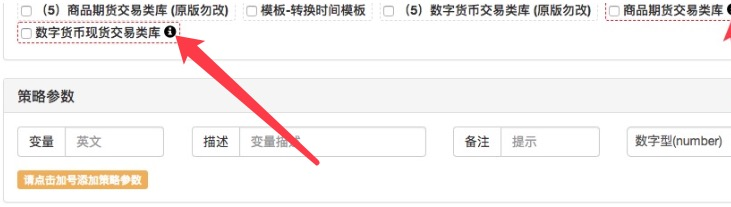点击箭头位置，也可以复制模板源码。

More

tiemuer 这个研究了一下好像如果你的单子如果一直没有成交，usdt被冻结，就会一直卡在那里，是不是这样

FangBei 有简单的python 模板的例子吗？

pixy3173 我觉得Cross = function(a, b)里面的内部逻辑有必要好好讲讲，反正看不懂呢 痛苦中

pixy3173 业界良心